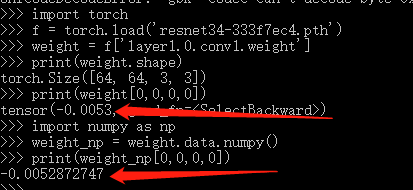# Why the values changed when converting a Variable to numpy?

I am trying to convert a parameter of resnet34 to numpy , but I found that the values would change after converting, as shown in the figure.
Why would this happen? What can I do to get precise values in numpy format?

( I am trying to get parameter in torch pretrained models and put them in tensorflow 1.x model , since I can’t find a pretrained resnet34 models in tensorflow1 afteing searching for several days. I am afraid this changed of values would affect the accuracy of the model. )

(I hate tensorflow…)This is most likely a printing issue and you could use `torch.set_printoptions(precision=15)` to increase the decimals.

1 Like